Your data analysis solution

# Mantel test in Excel tutorial

2017-10-20

This tutorial will help you set up and interpret a Mantel test used to test the association between distance matrices, in Excel using XLSTAT.
Not sure this is the statistical test you are looking for? Check out this guide.

## What is the Mantel test?

The Mantel test is useful to compute and test the linear correlation between two proximity matrices (dissimilarity or similarity). It is often used in Ecology to test if the dissimilarities between several sites, in terms abundancy of species on one side, and in terms of geophysical characteristics on the other side, can be related or not. Our example is in the field of genetics of populations.

## Dataset for running a Mantel test

An MS Excel sheet with both the data and the results can be downloaded by clicking here.

The data used in this tutorial correspond to a study where the researchers want to determine if the genetic distance between populations of insects in different regions of Africa is related to the geographical distance. The genetic distance is measured as a difference in gene frequencies among the various populations (there is only one gene of interest here). The geographical distance is based on distance in kilometers between the regions of interest, as well as on climatic distance.

## Setting up a Mantel test

To activate the Mantel test dialog box, start XLSTAT, then select the XLSTAT / Mantel test command, or click on the corresponding button of the XLSTAT toolbar (see below).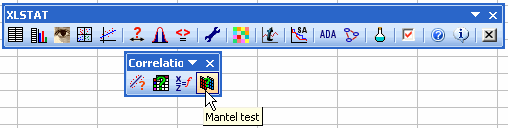Once you have clicked on the button, the dialog box appears. Then select the data corresponding the first dissimilarity matrix (A), and the data corresponding to the second dissimilarity matrix (B).

As the rows and columns labels of the matrices have been selected, we leave the Labels included option selected.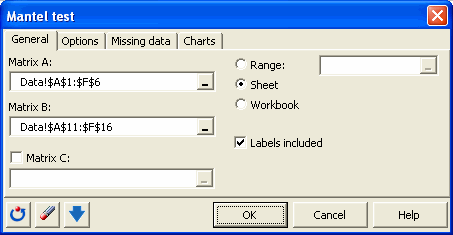In the Options tab we choose the two-tailed (r<>0) option in order to compute a two-tailed p-value.

The Exact p-value option is left activated. However, as the dimension of the matrices is too high (the limit is 4x4), the exact p-value won't be computed, and 10000 random permutations will be used to compute an estimated p-value.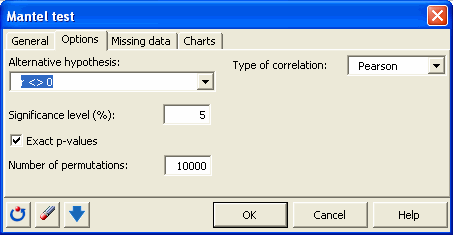After you have clicked on the OK button, the computations start and the results are displayed on a new Excel sheet.

## Interpreting the results of a Mantel test

The first result allows to visually verify if there is somehow a correlation between the proximities of the two matrices.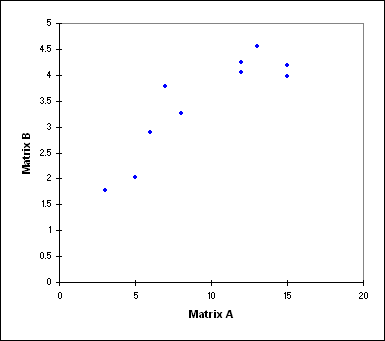The next table gives the Mantel r statistic, and the corresponding two-tailed p-value, obtained from the 10000 permutations. We can see here that as the conclusion confirms it, we should reject the null hypothesis that there is no correlation between the matrices. As a conclusion, we can consider that the the genetic and geographic distances are correlated.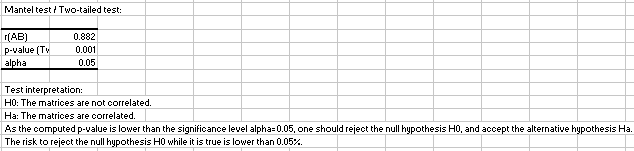A chart shows the distribution from which the p-value has been obtained.1c26995d494fb3061dd0ae8571ffc0a4@xlstat.desk-mail.com
https://cdn.desk.com/
false
desk